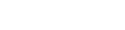• Home
• CLAT Maths Questions...

Author : Tanya Kaushal

January 17, 2023

SHARE

In CLAT, out of all five subjects, Maths holds the least weightage. Most of you might think of it as a skippable subject, but it can boost your marks. With the proper preparation, you can get all the questions correct to help you with a jump start.

The paper will ask 13-17 questions in the CLAT Maths section. This post provides an idea about the type of questions that can be asked from each topic and the difficulty level, essential CLAT Maths Questions with Answers provided for enhancing exam preparation.

This post shall take you through topic-wise Mathematics questions for CLAT Exam Preparation. Read through the entire post and practice the different maths questions asked in CLAT.

## What Type of Questions are Asked in the CLAT Maths Section?

As per the new pattern of CLAT, the entire question paper includes comprehension-based passages followed by 4-5 multiple-choice questions.

Solve these questions to understand the difficulty level of the exam and the questions asked.

1. In the maths section, you are given data to read and analyze. Following the data, you must answer the respective questions.
2. In one passage, you can come across different topic-based questions like profit and loss, discount, algebra, time, speed, and more.
3. The mathematics section included data interpretation-based questions from the previous year's CLAT Exam.

## How to Approach CLAT Maths Questions 2024?

Maths is one of the subjects in the CLAT Exam that holds a 10% weightage in the question paper. The below points explain tips on how to answer CLAT Maths Questions.

Though mathematics has less weightage in CLAT Exam, it is essential to be well-prepared for all topics to score full marks in this section.

Here are some essential tips and tricks to easily crack CLAT Elementary Mathematics 2024.

• Unlike previous years, the exam pattern is completely changed. The entire question paper includes passages and questions. Hence, it is essential to read the complete course thoroughly.
• Make sure to understand every line before proceeding with answering any question.
• You can solve the previous year's CLAT Question Papers to know which questions can be asked.
• As the question paper trend is changing each year, the exam conducting authority tests your ability to solve logical questions rather than just formula-based questions.
• While doing any calculations in the rough sheet during the exam, retain them.
• You may require the previous solution to answer the following question since the questions are comprehension-based.

## What are the Essential Tips to Solve CLAT Mathematics Questions 2024?

Make sure you have some tips and tricks to solve the questions in the Math section.

As the syllabus of CLAT Maths is vast, you need to study the concepts first and understand them before solving the questions.

1. Memorise the tables up to 25, squares, and cubes up to 20. This way, you can solve complex questions quickly.
2. Make sure to note down the formulas for each topic to help solve application-based problems.
3. Do not take too much time for this section in the examination. Try to be quick in applying the correct method and solving the problem.
4. Solving more and more questions is one of the best ways to improve your speed.

## Important CLAT Maths Questions

Though this section includes the least number of questions, it is important to practice all the topic-based questions regularly. If practised well, it is easy to score more marks.

You can follow CLAT Maths Preparation Tips designed by subject expertise from LegalEdge.

Let's understand CLAT Maths Questions as per the new pattern. Along with these important questions, you can solve CLAT Maths Quiz to analyze your preparation levels.

## CLAT Mathematics Questions 2024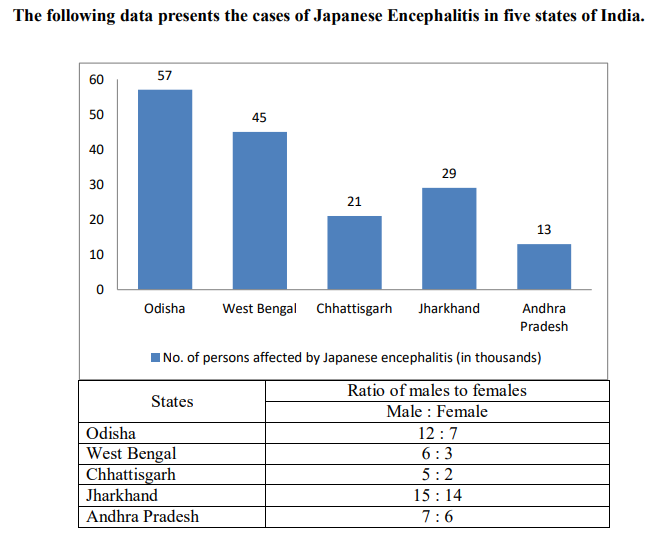Q. Number of females affected by Japanese encephalitis in West Bengal what percentage (up to 1 decimal) is more or less than that of females affected in Odisha?

a) 28.6 % less b) 29.7 % more c) 26.8 % less d) 25.8 % more

Q. What is the average number of males affected by Japanese encephalitis in all five states?

a) 9,000 b) 13,500 c) 18,400 d) 20,600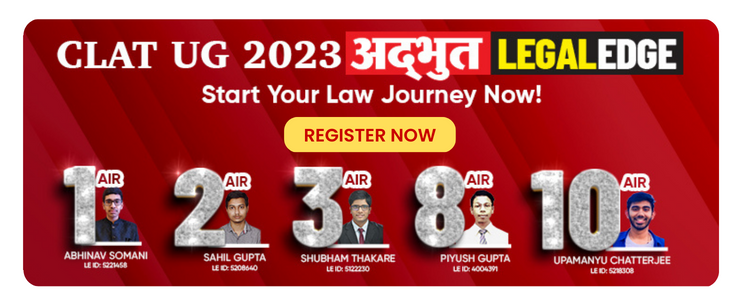Q. . If the total number of males affected by Japanese Encephalitis in other states is 87 % more than the average number of males in Odisha, West Bengal, Chhattisgarh and Jharkhand, the total number of males affected in India is:

a) 44,880 b) 1,40,880 c) 1,47,880 d) 68,880

Q. By what percentage is the average of males in Chhattisgarh, Jharkhand and Andhra Pradesh together more or less than the average of females in West Bengal, Jharkhand and Odisha together who are affected by Japanese encephalitis?

a) 22 b) 24 c) 26 d) 28

Q. What is the percentage (up to 1 decimal) of females affected by Japanese encephalitis‘ of the total affected population across five states?

a) 25.4 b) 36.7 c) 24.5 d) 37.6

A room having the dimensions mentioned in the chart below has a table, a bed, and a chair. There are three books kept on the table (side by side) and also a lamp having a radius, of 3.5 inches. Now, on the basis of the information given below, answer the following?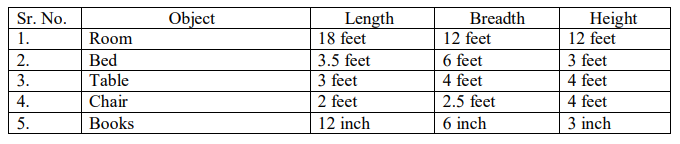Q. What would be the room area which is not covered by any object as mentioned above?

(a) 176.5 sq. ft. (b) 178 sq. ft. (c) 367 sq. ft. (d) 245.5 sq. ft.

Q. What area of the table is uncovered by any of the objects?

(a) 1656 sq. inch (b) 1617.52 sq. inch (c) 1473.52 sq. inch (d) 1512 sq. inch

Q. .How many tables are required to cover the room's floor surface completely?

(a) 18 (b) 11 (c) 21 (d) Data inadequate

Q. If the entire room is filled with books with the same dimensions as lying on the table, how many books are required?

(a) 2,00,736 (b) 20,736 (c) 11,736 (d) 17,522

Q. How many chairs can be put inside the room so as to cover the entire floor area of the room?

(a) 40 (b) 21 (c) 43 (d) 18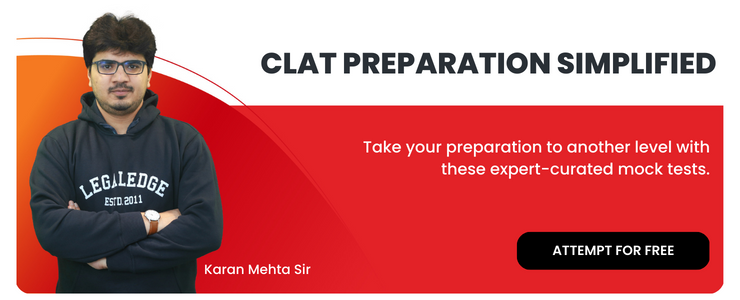## Sample CLAT Maths Questions

Here are a few sample questions from the quantitative aptitude section. During preparation, solving the CLAT Mock Test shall help you analyze your preparation levels and know different questions.

Q. The pie charts given here show some automobile parts manufactured by an automobile company at its Pune and Nagpur plants in the year 2009. Study the pie charts and answer questions. The number of total auto parts produced at both plants is the same.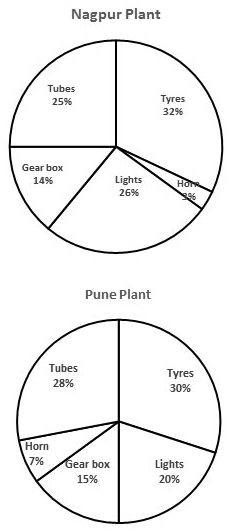If the Nagpur plant produced 800000 tyres, then the number of horns produced by it was:

A. 12000; B. 18500; C. 75000; D. 60000

Q. How many per cent more tubes were produced at the Pune plant than those Nagpur plants?

A. 14%; B. 12%; C. 8%; D. 3%

Q. The ratio of the number of horns produced at the Nagpur plant to that produced at the Pune plant is:

A. 3:7; B. 10:3; C. 7:3; D. 7:10

Q. The following table gives cost data of select stock prices on 3rd Dec 2019 in two markets, BSE of India and NQE of Kya Kya island. Closing stock refers to the price at the close of trading hours, and opening stock to the price at the beginning of the day. The currency of Kya Kya is #, and the exchange rate is # = Rs.11.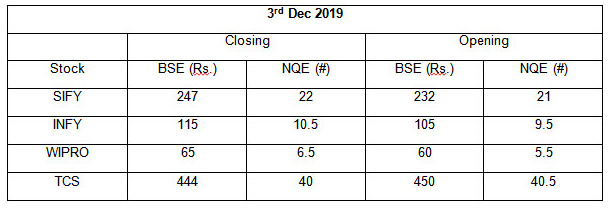Which share and on which market showed the highest per cent increase on 3rd Dec 2019?

A. SIFY and BSE; B. INFY and NQE; C. Wipro and NQE; D. TCS and NQE

Q. Arbitrage means buying in one market and selling simultaneously in another market. If Mr Ram was to buy a stock at the opening price on one exchange and be able to sell it at the opening price on the other exchange, on which share does he make the maximum % profit?

A. SIFY; B. INFY; C. Wipro; D. TCS

Q. In an M&A deal, SIFY is Purchased by its parent company SATYAM which purchases 15% of SIFY’s equity shares. Total SIFY’s equity shares are 1 million. How much does SATYAM pay in Rs. (approx) for the stake if 50% of its purchases were on BSE’s opening price and the balance on BSE’s closing price on 3rd Dec 2019?

A. 36 million; B. 3.5 million; C. 363 million; D. 217 million

Q. A bartender was different formulations for a cocktail party. He was considering a choice of 5 alternative cocktails. (A, B, C, D and E) which can be used in different proportions in the formulations. The table below gives the compositions of these cocktails. The table below gives the composition of these cocktails. The cost per unit of each of these is A – 150, B – 50, C – 200, D – 500, and E – 100.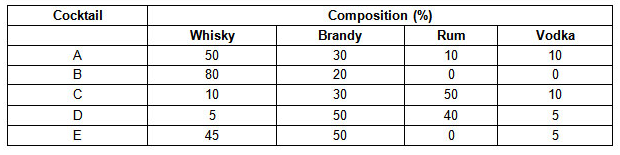If he wants to mix at least two of these cocktails to produce a drink containing 10% vodka and at least 30% brandy, how many ways can it be done?

A. One; B. Two; C. Three; D. Four

Q. Which of the following combinations gives a drink with the lowest cost per unit while having 10% rum and at least 30% brandy if it is to be formed by mixing two cocktails?

A. B and C; B. B and E; C. B and D; D. C and E

Q. What proportion should B, C and E be mixed to make a drink with at least 60% whisky at the lowest per-unit cost?

A. 2:1:3; B. 4:1:2; C. 2:1:4; D. 4:1:1

Q. If a drink containing at least 30% of each whisky and brandy and at least 5% of vodka and not more than 25% rum is to be made, then which one of the following combinations of equally mixed ingredients is feasible?

A. A and B; B. D and E; C. B and E; D. A and E

Q. All students of the Hyderabad International School have to learn exactly one of English and French as their first language. Given below is the proportion of Indian and International students and the proportion of students taking English or French at the school. The school has a total of 1600 students, 80% of whom are in the second section and the rest equally divided between Class 11 and 12.

 - Indian Students English Class 12 0.60 - Class 11 0.55 0.50 Secondary School - 0.55 Total 0.475 0.53

What is the percentage of Indian students in the secondary section?

A. 40; B. 45; C. 50; D. 55

Q. In class 12, twenty-five per cent of students who took English as their first language are Indian. What is the difference between the number of foreign students with English as their first language and Indian students with French as their first language?

A. Less than 16; B. 20; C. 24; D. 32

Q. What is the percentage of students in class 12 with English as their first language?

A. 40; B. 45; C. 50; D. 55

Q. In the secondary section, 50% of the Indian students had English as their first language. Which of the following statements is correct?

A. Except for Indian students with English as their first language, all other groups have the same number of students.; B. Except for Indian students with French as their first language, all other groups have the same number of students.; C. Except for foreign students with English as their first language, all other groups have the same number of students.; D. Except for foreign students with French as their first language, all other groups have the same number of students.

Q.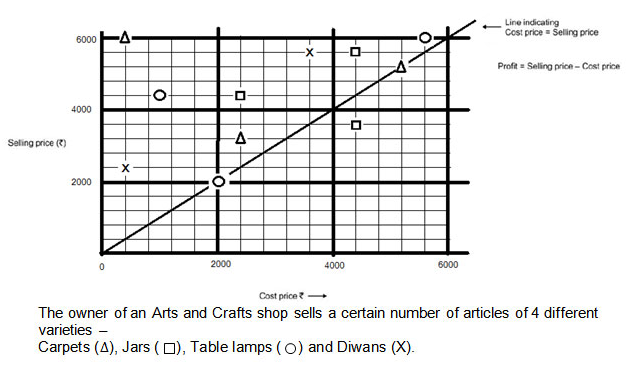Which variety of articles has the lowest average cost price?

A. Diwans; B. Table Lamps; C. Carpets; D. Jars

Q. Which variety of articles has the highest average selling price?

A. Diwans; B. Table Lamps; C. Carpets; D. Jars

Q. Article of which variety earned the highest profit?

A. Diwans; B. Table Lamps; C. Carpets; D. Jars

Q. Which variety has the lowest average profit?

A. Diwans; B. Table Lamps; C. Carpets; D. Jars

Q. For how many articles, the profit is more than 50% of the cost price?

A. 1; B. 2; C. 3; D. 4

Q. Study the following graph (Figure 4.8) carefully and answer the given questions. Gross Revenues and the Profit/Loss for HBL Limited (All figures in Rs crore)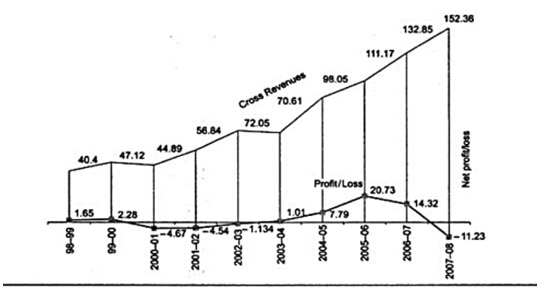The highest percentage of growth in total revenue was recorded in the:

A. 2000-01; B. 2001-02; C. 2002-03; D. 2004-05

Q. In which year was the net profit as a percentage of gross revenue at its second-highest level?

A. 2004-05; B. 2005-06; C. 2006-07; D. None of these

Q. For which of the following years were the trend gross revenue and net profit/loss dissimilar?

A. 2001-02; B. 2002-03; C. 2003-04; D. 2004-05

Q. The following pie chart shows the number of subscriptions generated for India Bonds from different categories of investors. Subscriptions Generated for India Bonds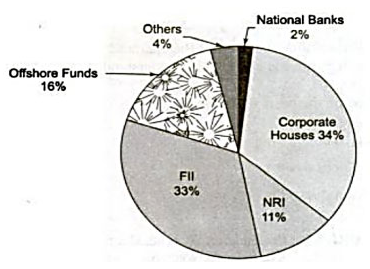If the investments by NRIs are Rs 4,000 crore, then the investment by corporate houses and FIIs together is:

A. 24,000 crore; B. 24,363 crore; C. 25,423.4 crore; D. 25,643.3 crore

Q. What percentage of the total investment is coming from either FIIs or NRIs?

A. 33%; B. 11%; C. 44%; D. 22%

Q. If the total investment other than by FII and corporate houses is Rs 335,000 crore, then the investment by NRIs and Offshore funds will be (approximately):

A. 274,100; B. 285,600; C. 293,000; D. Cannot be determined

Q. What is the approximate ratio of investment flows into India Bonds from NRIs to corporate houses?

A. 1:4; B. 1:3; C. 3:1; D. cannot be determined

Q. In the corporate sector, approximately how many degrees should be there in the central angle?

A. 120; B. 121; C. 122; D. 123

Q. If the total investment flows from FIIs were to be doubled in the next year, and the investment flows from all other sources had remained constant at their existing levels for this year, then what would be the proportion of FII investment in the total investment flows into India Bonds in the next year (approximately)?

A. 40%; B. 50%; C. 60%; D. 70%

### Previous Year CLAT Maths Questions

A room having the dimensions mentioned in the chart below has a table, a bed, and a chair. There are three books kept on the table (side by side) and also a lamp having a radius of 3.5 inches. Now, on the basis of the information given below, answer the following?

 Sr. No Object Length Breadth Height 1 Room 18 feet 12 feet 12 feet 2 Bed 3.5 feet 6 feet 3 feet 3 Table 3 feet 4 feet 4 feet 4 Chair 2 feet 2.5 feet 4 feet 5 Books 12 inch 6 inch 3 inch
1. What would be the area of the room which is not covered by any object as mentioned above? (a) 176.5 sq. ft. (b) 178 sq. ft. (c) 367 sq. ft. (d) 245.5 sq. ft.
2. What is the area of the table which is uncovered by any of the objects? (a) 1656 sq. inch (b) 1617.52 sq. inch (c) 1473.52 sq. inch (d) 1512 sq. inch
3. How many tables are required to cover the floor surface of the room completely? (a) 18 (b) 11 (c) 21 (d) Data inadequate.
4. If the entire room is to be filled with books having the same dimensions as lying on the table, then how many books are required? (a) 2,00,736 (b) 20,736 (c) 11,736 (d) 17,522
5. How many chairs can be put inside the room so as to cover the entire floor area of the room? (a) 40 (b) 21 (c) 43 (d) 18

In the figure given below, a goat is tied to a pole (at point O) which is the centre of the semi-circular plot. The area of the plot is equal to its perimeter. BO is the length of the rope through which the goat is tied to the pole. Answer to the closest decimal.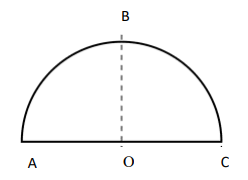1. The length of the rope is a. 2 + π b. 2 + π/2 c. 2 + 2/ π d. 2
2. The area of the plot is a. 18.13 b. 21.83 c. 28.13 d. 11.83
3. If a triangle is created by joining points A, B, and C. What will be the length of AB? a. 3.73 b. 7.33 c. 1.86 d. Can‘t be calculated with the given data
4. What will be the area of the triangle ABC? a. 6.96 b. 9.66 c. 3.66 d. Can‘t be calculated with the given data
5. What will be the perimeter of the triangle ABC? a. 40.4 b. 30.3 c. 20.2 d. 10.1

Following the expert tips will help you to prepare better for the CLAT Maths 2024. Begin your preparation by studying from the right books, which cover all the concepts and topics according to the latest exam pattern. Make sure you know the detailed paper pattern and the latest CLAT exam syllabus before starting your preparation.

To prepare for the CLAT Exam, you must choose the books that are best to study. Some of the best books for preparing for the CLAT Maths Section are: Quantitative Aptitude by Pearson, First Track Objective Arithmetic by Arihant, 30 days wonder for Maths S. Chand, Legal Aptitude (CLAT) by Samiksha Gupta and R.K Gupta.

Some of the general preparation tips to crack the Maths section are: Try to understand the concepts of the topics. Do not go cramming. Do not try to get into some new topics at the end day of preparation. Revise the old topics. Go through the formulae, and try practising them. During preparation, check shortcuts too.

You can easily improve your speed and accuracy in the CLAT Exam by solving as many sample papers as possible. Practising mock tests would improve your time management skills and problem-solving techniques. So, it is advisable to solve mock tests daily.
• Home
• CLAT Maths Questions...

Author : Tanya Kaushal

Updated On : January 17, 2023

SHARE

In CLAT, out of all five subjects, Maths holds the least weightage. Most of you might think of it as a skippable subject, but it can boost your marks. With the proper preparation, you can get all the questions correct to help you with a jump start.

The paper will ask 13-17 questions in the CLAT Maths section. This post provides an idea about the type of questions that can be asked from each topic and the difficulty level, essential CLAT Maths Questions with Answers provided for enhancing exam preparation.

This post shall take you through topic-wise Mathematics questions for CLAT Exam Preparation. Read through the entire post and practice the different maths questions asked in CLAT.

## What Type of Questions are Asked in the CLAT Maths Section?

As per the new pattern of CLAT, the entire question paper includes comprehension-based passages followed by 4-5 multiple-choice questions.

Solve these questions to understand the difficulty level of the exam and the questions asked.

1. In the maths section, you are given data to read and analyze. Following the data, you must answer the respective questions.
2. In one passage, you can come across different topic-based questions like profit and loss, discount, algebra, time, speed, and more.
3. The mathematics section included data interpretation-based questions from the previous year's CLAT Exam.

## How to Approach CLAT Maths Questions 2024?

Maths is one of the subjects in the CLAT Exam that holds a 10% weightage in the question paper. The below points explain tips on how to answer CLAT Maths Questions.

Though mathematics has less weightage in CLAT Exam, it is essential to be well-prepared for all topics to score full marks in this section.

Here are some essential tips and tricks to easily crack CLAT Elementary Mathematics 2024.

• Unlike previous years, the exam pattern is completely changed. The entire question paper includes passages and questions. Hence, it is essential to read the complete course thoroughly.
• Make sure to understand every line before proceeding with answering any question.
• You can solve the previous year's CLAT Question Papers to know which questions can be asked.
• As the question paper trend is changing each year, the exam conducting authority tests your ability to solve logical questions rather than just formula-based questions.
• While doing any calculations in the rough sheet during the exam, retain them.
• You may require the previous solution to answer the following question since the questions are comprehension-based.

## What are the Essential Tips to Solve CLAT Mathematics Questions 2024?

Make sure you have some tips and tricks to solve the questions in the Math section.

As the syllabus of CLAT Maths is vast, you need to study the concepts first and understand them before solving the questions.

1. Memorise the tables up to 25, squares, and cubes up to 20. This way, you can solve complex questions quickly.
2. Make sure to note down the formulas for each topic to help solve application-based problems.
3. Do not take too much time for this section in the examination. Try to be quick in applying the correct method and solving the problem.
4. Solving more and more questions is one of the best ways to improve your speed.

## Important CLAT Maths Questions

Though this section includes the least number of questions, it is important to practice all the topic-based questions regularly. If practised well, it is easy to score more marks.

You can follow CLAT Maths Preparation Tips designed by subject expertise from LegalEdge.

Let's understand CLAT Maths Questions as per the new pattern. Along with these important questions, you can solve CLAT Maths Quiz to analyze your preparation levels.

## CLAT Mathematics Questions 2024Q. Number of females affected by Japanese encephalitis in West Bengal what percentage (up to 1 decimal) is more or less than that of females affected in Odisha?

a) 28.6 % less b) 29.7 % more c) 26.8 % less d) 25.8 % more

Q. What is the average number of males affected by Japanese encephalitis in all five states?

a) 9,000 b) 13,500 c) 18,400 d) 20,600Q. . If the total number of males affected by Japanese Encephalitis in other states is 87 % more than the average number of males in Odisha, West Bengal, Chhattisgarh and Jharkhand, the total number of males affected in India is:

a) 44,880 b) 1,40,880 c) 1,47,880 d) 68,880

Q. By what percentage is the average of males in Chhattisgarh, Jharkhand and Andhra Pradesh together more or less than the average of females in West Bengal, Jharkhand and Odisha together who are affected by Japanese encephalitis?

a) 22 b) 24 c) 26 d) 28

Q. What is the percentage (up to 1 decimal) of females affected by Japanese encephalitis‘ of the total affected population across five states?

a) 25.4 b) 36.7 c) 24.5 d) 37.6

A room having the dimensions mentioned in the chart below has a table, a bed, and a chair. There are three books kept on the table (side by side) and also a lamp having a radius, of 3.5 inches. Now, on the basis of the information given below, answer the following?Q. What would be the room area which is not covered by any object as mentioned above?

(a) 176.5 sq. ft. (b) 178 sq. ft. (c) 367 sq. ft. (d) 245.5 sq. ft.

Q. What area of the table is uncovered by any of the objects?

(a) 1656 sq. inch (b) 1617.52 sq. inch (c) 1473.52 sq. inch (d) 1512 sq. inch

Q. .How many tables are required to cover the room's floor surface completely?

(a) 18 (b) 11 (c) 21 (d) Data inadequate

Q. If the entire room is filled with books with the same dimensions as lying on the table, how many books are required?

(a) 2,00,736 (b) 20,736 (c) 11,736 (d) 17,522

Q. How many chairs can be put inside the room so as to cover the entire floor area of the room?

(a) 40 (b) 21 (c) 43 (d) 18## Sample CLAT Maths Questions

Here are a few sample questions from the quantitative aptitude section. During preparation, solving the CLAT Mock Test shall help you analyze your preparation levels and know different questions.

Q. The pie charts given here show some automobile parts manufactured by an automobile company at its Pune and Nagpur plants in the year 2009. Study the pie charts and answer questions. The number of total auto parts produced at both plants is the same.If the Nagpur plant produced 800000 tyres, then the number of horns produced by it was:

A. 12000; B. 18500; C. 75000; D. 60000

Q. How many per cent more tubes were produced at the Pune plant than those Nagpur plants?

A. 14%; B. 12%; C. 8%; D. 3%

Q. The ratio of the number of horns produced at the Nagpur plant to that produced at the Pune plant is:

A. 3:7; B. 10:3; C. 7:3; D. 7:10

Q. The following table gives cost data of select stock prices on 3rd Dec 2019 in two markets, BSE of India and NQE of Kya Kya island. Closing stock refers to the price at the close of trading hours, and opening stock to the price at the beginning of the day. The currency of Kya Kya is #, and the exchange rate is # = Rs.11.Which share and on which market showed the highest per cent increase on 3rd Dec 2019?

A. SIFY and BSE; B. INFY and NQE; C. Wipro and NQE; D. TCS and NQE

Q. Arbitrage means buying in one market and selling simultaneously in another market. If Mr Ram was to buy a stock at the opening price on one exchange and be able to sell it at the opening price on the other exchange, on which share does he make the maximum % profit?

A. SIFY; B. INFY; C. Wipro; D. TCS

Q. In an M&A deal, SIFY is Purchased by its parent company SATYAM which purchases 15% of SIFY’s equity shares. Total SIFY’s equity shares are 1 million. How much does SATYAM pay in Rs. (approx) for the stake if 50% of its purchases were on BSE’s opening price and the balance on BSE’s closing price on 3rd Dec 2019?

A. 36 million; B. 3.5 million; C. 363 million; D. 217 million

Q. A bartender was different formulations for a cocktail party. He was considering a choice of 5 alternative cocktails. (A, B, C, D and E) which can be used in different proportions in the formulations. The table below gives the compositions of these cocktails. The table below gives the composition of these cocktails. The cost per unit of each of these is A – 150, B – 50, C – 200, D – 500, and E – 100.If he wants to mix at least two of these cocktails to produce a drink containing 10% vodka and at least 30% brandy, how many ways can it be done?

A. One; B. Two; C. Three; D. Four

Q. Which of the following combinations gives a drink with the lowest cost per unit while having 10% rum and at least 30% brandy if it is to be formed by mixing two cocktails?

A. B and C; B. B and E; C. B and D; D. C and E

Q. What proportion should B, C and E be mixed to make a drink with at least 60% whisky at the lowest per-unit cost?

A. 2:1:3; B. 4:1:2; C. 2:1:4; D. 4:1:1

Q. If a drink containing at least 30% of each whisky and brandy and at least 5% of vodka and not more than 25% rum is to be made, then which one of the following combinations of equally mixed ingredients is feasible?

A. A and B; B. D and E; C. B and E; D. A and E

Q. All students of the Hyderabad International School have to learn exactly one of English and French as their first language. Given below is the proportion of Indian and International students and the proportion of students taking English or French at the school. The school has a total of 1600 students, 80% of whom are in the second section and the rest equally divided between Class 11 and 12.

 - Indian Students English Class 12 0.60 - Class 11 0.55 0.50 Secondary School - 0.55 Total 0.475 0.53

What is the percentage of Indian students in the secondary section?

A. 40; B. 45; C. 50; D. 55

Q. In class 12, twenty-five per cent of students who took English as their first language are Indian. What is the difference between the number of foreign students with English as their first language and Indian students with French as their first language?

A. Less than 16; B. 20; C. 24; D. 32

Q. What is the percentage of students in class 12 with English as their first language?

A. 40; B. 45; C. 50; D. 55

Q. In the secondary section, 50% of the Indian students had English as their first language. Which of the following statements is correct?

A. Except for Indian students with English as their first language, all other groups have the same number of students.; B. Except for Indian students with French as their first language, all other groups have the same number of students.; C. Except for foreign students with English as their first language, all other groups have the same number of students.; D. Except for foreign students with French as their first language, all other groups have the same number of students.

Q.Which variety of articles has the lowest average cost price?

A. Diwans; B. Table Lamps; C. Carpets; D. Jars

Q. Which variety of articles has the highest average selling price?

A. Diwans; B. Table Lamps; C. Carpets; D. Jars

Q. Article of which variety earned the highest profit?

A. Diwans; B. Table Lamps; C. Carpets; D. Jars

Q. Which variety has the lowest average profit?

A. Diwans; B. Table Lamps; C. Carpets; D. Jars

Q. For how many articles, the profit is more than 50% of the cost price?

A. 1; B. 2; C. 3; D. 4

Q. Study the following graph (Figure 4.8) carefully and answer the given questions. Gross Revenues and the Profit/Loss for HBL Limited (All figures in Rs crore)The highest percentage of growth in total revenue was recorded in the:

A. 2000-01; B. 2001-02; C. 2002-03; D. 2004-05

Q. In which year was the net profit as a percentage of gross revenue at its second-highest level?

A. 2004-05; B. 2005-06; C. 2006-07; D. None of these

Q. For which of the following years were the trend gross revenue and net profit/loss dissimilar?

A. 2001-02; B. 2002-03; C. 2003-04; D. 2004-05

Q. The following pie chart shows the number of subscriptions generated for India Bonds from different categories of investors. Subscriptions Generated for India BondsIf the investments by NRIs are Rs 4,000 crore, then the investment by corporate houses and FIIs together is:

A. 24,000 crore; B. 24,363 crore; C. 25,423.4 crore; D. 25,643.3 crore

Q. What percentage of the total investment is coming from either FIIs or NRIs?

A. 33%; B. 11%; C. 44%; D. 22%

Q. If the total investment other than by FII and corporate houses is Rs 335,000 crore, then the investment by NRIs and Offshore funds will be (approximately):

A. 274,100; B. 285,600; C. 293,000; D. Cannot be determined

Q. What is the approximate ratio of investment flows into India Bonds from NRIs to corporate houses?

A. 1:4; B. 1:3; C. 3:1; D. cannot be determined

Q. In the corporate sector, approximately how many degrees should be there in the central angle?

A. 120; B. 121; C. 122; D. 123

Q. If the total investment flows from FIIs were to be doubled in the next year, and the investment flows from all other sources had remained constant at their existing levels for this year, then what would be the proportion of FII investment in the total investment flows into India Bonds in the next year (approximately)?

A. 40%; B. 50%; C. 60%; D. 70%

### Previous Year CLAT Maths Questions

A room having the dimensions mentioned in the chart below has a table, a bed, and a chair. There are three books kept on the table (side by side) and also a lamp having a radius of 3.5 inches. Now, on the basis of the information given below, answer the following?

 Sr. No Object Length Breadth Height 1 Room 18 feet 12 feet 12 feet 2 Bed 3.5 feet 6 feet 3 feet 3 Table 3 feet 4 feet 4 feet 4 Chair 2 feet 2.5 feet 4 feet 5 Books 12 inch 6 inch 3 inch
1. What would be the area of the room which is not covered by any object as mentioned above? (a) 176.5 sq. ft. (b) 178 sq. ft. (c) 367 sq. ft. (d) 245.5 sq. ft.
2. What is the area of the table which is uncovered by any of the objects? (a) 1656 sq. inch (b) 1617.52 sq. inch (c) 1473.52 sq. inch (d) 1512 sq. inch
3. How many tables are required to cover the floor surface of the room completely? (a) 18 (b) 11 (c) 21 (d) Data inadequate.
4. If the entire room is to be filled with books having the same dimensions as lying on the table, then how many books are required? (a) 2,00,736 (b) 20,736 (c) 11,736 (d) 17,522
5. How many chairs can be put inside the room so as to cover the entire floor area of the room? (a) 40 (b) 21 (c) 43 (d) 18

In the figure given below, a goat is tied to a pole (at point O) which is the centre of the semi-circular plot. The area of the plot is equal to its perimeter. BO is the length of the rope through which the goat is tied to the pole. Answer to the closest decimal.1. The length of the rope is a. 2 + π b. 2 + π/2 c. 2 + 2/ π d. 2
2. The area of the plot is a. 18.13 b. 21.83 c. 28.13 d. 11.83
3. If a triangle is created by joining points A, B, and C. What will be the length of AB? a. 3.73 b. 7.33 c. 1.86 d. Can‘t be calculated with the given data
4. What will be the area of the triangle ABC? a. 6.96 b. 9.66 c. 3.66 d. Can‘t be calculated with the given data
5. What will be the perimeter of the triangle ABC? a. 40.4 b. 30.3 c. 20.2 d. 10.1

Following the expert tips will help you to prepare better for the CLAT Maths 2024. Begin your preparation by studying from the right books, which cover all the concepts and topics according to the latest exam pattern. Make sure you know the detailed paper pattern and the latest CLAT exam syllabus before starting your preparation.

To prepare for the CLAT Exam, you must choose the books that are best to study. Some of the best books for preparing for the CLAT Maths Section are: Quantitative Aptitude by Pearson, First Track Objective Arithmetic by Arihant, 30 days wonder for Maths S. Chand, Legal Aptitude (CLAT) by Samiksha Gupta and R.K Gupta.

Some of the general preparation tips to crack the Maths section are: Try to understand the concepts of the topics. Do not go cramming. Do not try to get into some new topics at the end day of preparation. Revise the old topics. Go through the formulae, and try practising them. During preparation, check shortcuts too.

You can easily improve your speed and accuracy in the CLAT Exam by solving as many sample papers as possible. Practising mock tests would improve your time management skills and problem-solving techniques. So, it is advisable to solve mock tests daily.